Start typing, then use the up and down arrows to select an option from the list.# Osmolarity Example 2

Jules Bruno
143views
1
now remember method to we can use OSB Olara t equals number of ions times the polarity of the compound Here In this example, it says What is the concentration off hydroxide ions in a 0.350 Mueller solution off gallium hydroxide which has a formula of G a O. H. Three. All right, so we need to find the Osma clarity of hydroxide ions. So it's morality of O. H minus ions. It equals the number of hydroxide ions. If we take a look here at the formula, we see that there's a three here meaning that there are three hydroxide within this parentheses, so that would be three times now. The polarity of the original compound gallium hydroxide as a compound has a polarity up 0.350 Moeller. So now just do three times that value. And when we do that, we get 1.5 Moeller as the Osma polarity off hydroxide ions. So it's a simple is that if you know the polarity of the compound overall, use that to help you find the odd more clarity of any one of its ions.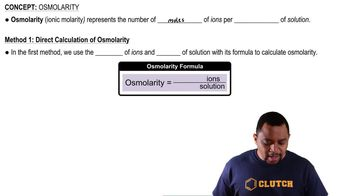01:10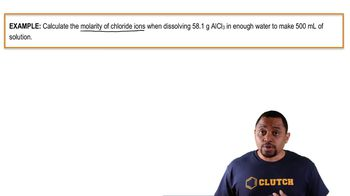02:55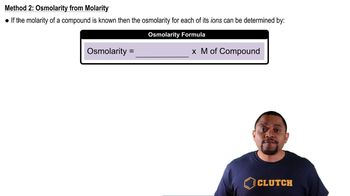00:37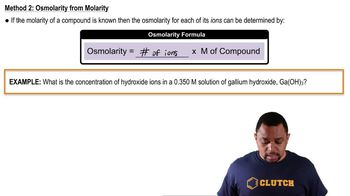01:16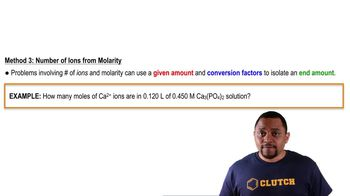02:18